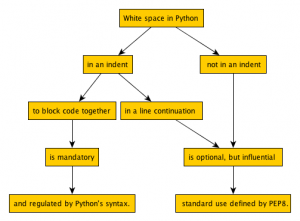Mind Map of White Space in Python

Nov 14, 2012 • Alex Viana

White space is a trivial but powerful concept in Python. It fits nicely into “a half-dozen boxes and arrows”, but can be a useful tool to getting scientist to thinking about the readability of their code.

Mind MapAn Illustrative Example

Here is a weird but functional piece of Python code:

for i in range(100):
result = (((i+5) * (i*2)) / 500) ** (1/2)
if result < 100:
print 'small'
else:
print 'big
save_result_to_a_file(this='', takes='', too='',
many='', arguments='')

What happens here? The for loop generates a list of 100 integers from 0 to 99. Then a math formula is computed and the result is assigned to a variable called result. If result is less than 100, ‘small’ is printed. Otherwise, ‘big’ is printed. Finally, a function, which we assume is defined elsewhere, called save_result_to_file, saves each instance of result to the file. Let’s look at the role white space plays in this code block.

Some of this white space is essential, it blocks control statements together. Changing that white space would affect the functionality of the code, in some cases breaking it and in other cases changing the output. But some of the white space is optional and Python is very flexible about how you use white space. There are standard practices about how to use optional white space, defined by a document called PEP8. This is similar to a style guide for written English such as Strunk and White.

You can always “break the rules” about using optional white space, but you should be aware that there are conventions and think about how breaking them might affect other users of your code. Here is a counter-example with non-standard optional white space. It will still run, and produce the same result, but it will be less readable to anyone familiar with Python.

for i in range( 100 ) :
result = ( ( ( i + 5 ) * ( i * 2 ) ) / 500 ) *                     ( 1/2 )
if result<100  :
print 'small'
else              :
print 'big'
save_result_to_a_file(this='',takes='',too='',many='',
arguments='')

Now, this isn’t too hard to read but the problems become worse the more complicated the code and is the less familiar the user is with it.

Concept reinforcement examples:

Example 1:

Indentation (typically 4 spaces) is used to block code together such as in control statements like an if statements or a for loops. Code that immediately follows a control statement and is indented relative to the control statement is executed when the control statement is triggered. For example:

for i in range(5):
print i
print 'done'

gives:

0
1
2
3
4
done

Now change the white space so that done is printed after every number.

Example 2

We just saw how indentation can change when code is executed. When control statements are nested each block of code to be executed needs to be indented relative to its control statement, even if it’s already inside another control statement. For example:

for number in [1, 2]:
print number
for letter in ['a', 'b']:
print letter

gives:

1
2
a
b

while

for number in [1, 2]:
print number
for letter in ['a', 'b']:
print letter

gives:

1
a
b
2
a
b

Apply that to this code

for i in range(5):
print i
if i == 4:
print 'Found a 4!'

and change the use of white space so that the code prints the following:

1
2
3
4
'Found a 4!'

Example 3

Muti-part control statements such as if/else statements should be aligned so that the control statements are equally indented. The code to be executed should still be indented relative to its control statement. This is similar to what we saw in Example 2. Change the whitespace in the following code:

for i in range(5):
if i == 5:
print 'Found a 4!'
else:
print 'Not a 4'

so that it gives:

Not a 4
Not a 4
Not a 4
Not a 4
Found a 4!

Example 4

Not all white space will change how your code runs. In fact python is very flexible about where it allows you to place white space. But, non-standard use of white space can make it harder to read your code. Just like you can write very confusing English that is still grammatically correct.

One recommendation is to avoid extra white space inside of parentheses, brackets or braces.

('yes')
( 'no' )

The same for immediately before a comma, semicolon, or colon.

'this' , 'is' , 'bad'
'this', 'is', 'good'

Remove the extra white space in the line below. Remember while it is correct Python as written it is not the recommended use.

animals = { 'cats' : 4 ,  'dogs' : 1 }

Example 5:

It is recommended to put white space around operators such as comparisons (==), assignments (=), Booleans (and), and math operators (+). Add white space to fix the following two lines

if question==False:
print 1*5

Example 6:

For long mathematical expressions you can group the higher order operations leaving spaces around the lower order operations. For example:

(1*5) + (2*6)

Play with the following line of math. See if you can find a more condensed and readable version.

( ( 1 + 2 ) * ( 3 - 4 ) / (7 + 8 ) ) ** ( 9 / 10 )

Extension Example:

White space is also used to block together functions and classes. Let’s look at a more complicated piece of code to illustrate this. If classes are unfamiliar to you just focus on the concepts we just learned about white space.

class Circle(object):